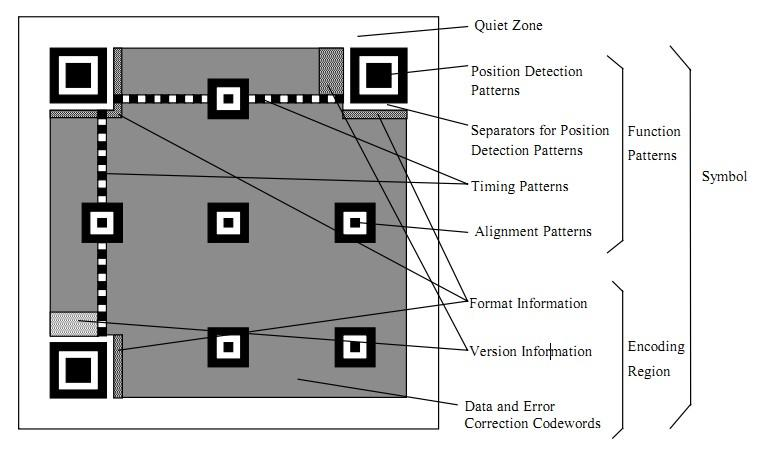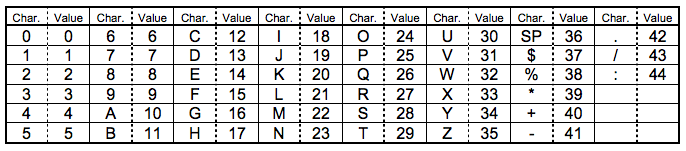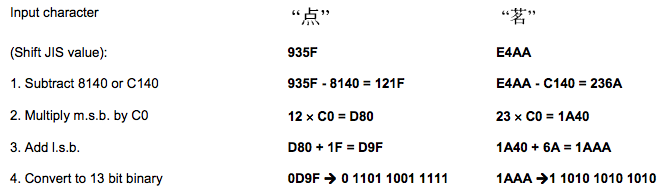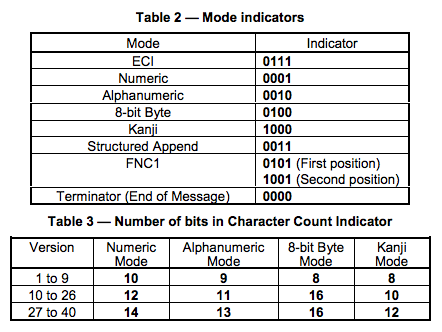# QR CODE Generation Details and Principles

QR code, also known as QR Code, QR full name Quick Response, is a popular encoding method on mobile devices in recent years. It can store more information and more data than traditional Bar Code barcodes. Type: For example: characters, numbers, Japanese, Chinese, etc. I learned about the details of the QR code image generation in the past two days. I feel that this gadget is a cryptographic algorithm. Write this article here and expose it. For those who are good at learning to learn together.

#### Basic knowledge

First, let's talk about the QR code for a total of 40 sizes. The official version is Version. Version 1 is a 21 x 21 matrix, Version 2 is a 25 x 25 matrix, and Version 3 is a size of 29. Each additional version adds 4 dimensions. The formula is: (V-1)*4 + 21 ( V is the version number) The highest Version 40, (40-1) * 4 + 21 = 177, so the highest is 177 x 177 square.

Let's take a look at a sample of a QR code:#### Positioning pattern

• Position Detection Pattern is a positioning pattern used to mark the size of a rectangle of a QR code. The three positioning patterns have white edges called Separators for Postion Detection Patterns. The reason why three instead of four means that three can identify a rectangle.
• Timing Patterns is also used for positioning. The reason is that the QR code has 40 sizes. If the size is too large, you need to have a standard line. Otherwise, it may be broomed when scanning.
• Alignment Patterns Only two-dimensional codes of Version 2 or higher (including Version 2) need this stuff, and are also used for positioning.

#### Functional data

• Format Information exists in all dimensions for storing some formatted data.
• Version Information Above >= Version 7, you need to reserve two 3 x 6 areas to store some version information.

#### Data code and error correction code

• In addition to the above, the rest of the place stores the Data Code data code and the Error Correction Code error correction code.

### Data encoding

Let's talk about data encoding first. The QR code supports the following encoding:

Numeric mode:  digital encoding, from 0 to 9. If the number of numbers to be encoded is not a multiple of 3, then the last remaining 1 or 2 digits will be converted to 4 or 7 bits, and the other 3 digits will be programmed into 10, 12, 14 bits. How long does it take to see the size of the QR code (there is a table below, Table 3 illustrates this)

Alphanumeric mode:  character encoding. Includes 0-9, uppercase A to Z (no lowercase), and the symbol \$% * + – . / : including spaces. These characters are mapped into a character index table. As shown below: (where SP is a space, Char is a character, Value is its index value) The process of encoding is to group the characters into two, and then convert to the 45-digit of the following table, and then convert to 11-bit binary, if the last If there is a single order, it will be converted into a 6bit binary. The encoding mode and the number of characters need to be programmed into 9, 11 or 13 binary according to different Version sizes (Table 3 in the following table).Byte mode , byte encoding, can be ISO-8859-1 characters from 0-255. Some QR code scanners can automatically detect if they are UTF-8 encoding.

Kanji mode  This is Japanese encoding and is also double-byte encoding. Similarly, it can also be used for Chinese encoding. The encoding of Japanese and Chinese characters will be subtracted from a value. For example, the characters in 0X8140 to 0X9FFC will be subtracted from 8140, the characters in 0XE040 to 0XEBBF will be subtracted from 0XC140, then the first two hexadecimal digits of the result will be multiplied by 0XC0, and then the last two 16 The digit is converted to a 13-bit encoding. The following example shows:Extended Channel Interpretation (ECI) mode is  primarily used for special character sets. Not all scanners support this encoding.

Structured Append mode is  used for mixed encoding, that is, this QR code contains multiple encoding formats.

The encoding method of FNC1 mode is mainly used for some special industries or industries. Such as GS1 barcodes and the like.

For the sake of simplicity, the latter three will not be discussed in this article.

In the following two tables,

• Table 2 is the "number" of each encoding format, and this thing is written in Format Information. Note: Chinese is 1101
• Table 3 shows the two-dimensional code for different versions (sizes), for numbers, characters, bytes, and Kanji modes, for a single encoded binary number of bits. (In the specification of the QR code, there are various coding specification tables, which will be mentioned later)Let's look at a few examples.

### Example 1: Digital Code

In the case of Version 1, the error correction level is H, code: 01234567
1. Divide the above figures into three groups: 012 345 67
2. Convert them to binary: 012 to 0000001100; 345 to 0101011001; 67 to 1000011.
3. String these three binaryes together: 0000001100 0101011001 1000011
4. Convert the number of digits to binary (version 1-H is 10 bits): The binary of 8 digits is 0000001000
5.  Add the digitally encoded flag 0001 and the code of step 4 to the front: 0001 0000001000 0000001100 0101011001 1000011

### Example 2: Character Encoding

In the case of Version 1, the error correction level is H, code: AC-42
1. Find the index of the five words AC-42 from the character index table (10,12,41,4,2)
2. Two-two grouping: (10,12) (41,4) (2)
3. Convert each group to 11bits of binary:
(10,12) 10*45+12 equals 462 to 00111001110
(41,4) 41*45+4 equals 1849 to 11100111001
(2) equals 2 to 000010
4. Connect these binaries: 00111001110 11100111001 000010
5. Convert the number of characters to binary (Version 1-H is 9 bits): 5 characters, 5 to 000000101
6. Add the code number 0010 and the number of the 5th step to the head: 0010 000000101 00111001110 11100111001 000010